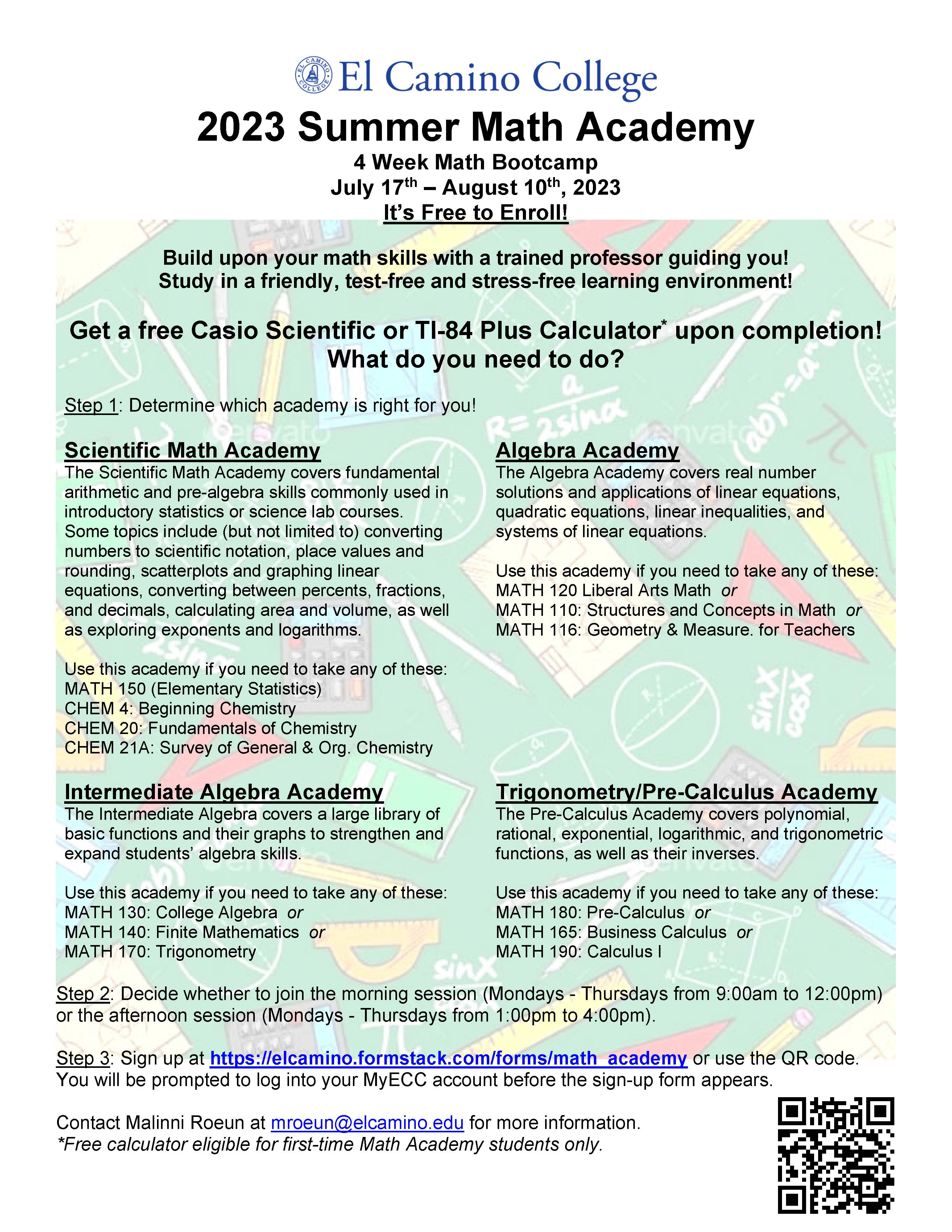Study in a friendly, test-free, stress-free, and non-college credit environment!

The Math Academy provides students with a refresher of mathematics skills to increase chances for success in their science or transfer-level courses.  Math Academy is not an event for determining math placement.  Math Academy is offered every winter and summer sessions four days a week for three hours per day.  Study in a friendly, test-free, stress-free, and non-college credit environment!

### Benefits

Students receive all of the supplies for the course free.  Plus, there is no fee to take the course.

• A free Texas Instrument (TI-84 Plus) Calculator or Casio fx-260 Solar II to keep and use in your science or transfer-level math course (students who repeat the course are ineligible for the calculator);
• A seal-stamped certificate of completion; and
• A personal congratulations from the dean.

Step 1: Determine which academy is right for you!

Scientific Math Academy (for science courses)

• The Scientific Math Academy covers fundamental arithmetic and pre-algebra skills commonly used in introductory statistics or science lab courses.  Some topics include (but not limited to) converting numbers to scientific notation, place values and rounding, scatterplots and graphing linear equations, converting between percents, fractions, and decimals, calculating area and volume, as well as exploring exponents and logarithms.  Use this academy if you need to take any of these courses:
• MATH 150: Elementary Statistics  or
• CHEM 4: Beginning Chemistry  or
• CHEM 20: Fundamentals of Chemistry  or
• CHEM 21A: Survey of General & Organic Chemistry

• The Algebra Academy covers real number solutions and applications of linear equations, quadratic equations, linear inequalities, and systems of linear equations.  Use this academy if you need to take any of these courses:
• MATH 120: Liberal Arts Mathematics  or
• MATH 110: Structures and Concepts in Mathematics  or
• MATH 116: Geometry & Measurement for Prospective Elementary School Teachers

• The Intermediate Algebra covers a large library of basic functions and their graphs to strengthen and expand students’ algebra skills.  Use this academy if you need to take any of these courses:
• MATH 130: College Algebra  or
• MATH 140: Finite Mathematics  or
• MATH 170: Trigonometry

• The Pre-Calculus Academy covers polynomial, rational, exponential, logarithmic, and trigonometric functions, as well as their inverses.  Use this academy if you need to take any of these courses:
• MATH 165: Calculus for Business & Social Sciences  or
• MATH 190: Calculus I

Step 2: Decide whether you want to participate in the Morning Group (Mondays through Thursdays from 9:00am - 12:00pm) or the Afternoon Group (Mondays through Thursdays from 1:00pm - 4:00pm).